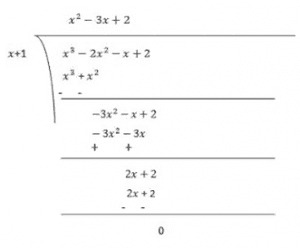Guru

# Factorize: (i) x3–2×2–x+2 Q.5(1)

• 0

Give me the best and simple way for solving the question from class 9th of Polynomials chapter. Give me the simple way for solving this problem Factorize: (i) x3–2×2–x+2

Share

1. Let p(x) = x3–2x2–x+2

Factors of 2 are ±1 and ± 2

Now,

p(x) = x3–2x2–x+2

p(−1) = (−1)3–2(−1)2–(−1)+2

= −1−2+1+2

= 0

Therefore, (x+1) is the factor of p(x)Now, Dividend = Divisor × Quotient + Remainder

(x+1)(x2–3x+2) = (x+1)(x2–x–2x+2)

= (x+1)(x(x−1)−2(x−1))

= (x+1)(x−1)(x-2)

• 0